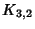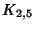## Complete Bipartite GraphA Bipartite Graph (i.e., a set of Vertices decomposed into two disjoint sets such that there are no two Vertices within the same set are adjacent) such that every pair of Vertices in the two sets are adjacent. If there areandVertices in the two sets, the complete bipartite graph (sometimes also called a Complete Bigraph) is denoted. The above figures showand.

See also Bipartite Graph, Complete Graph, Complete k-Partite Graph, k-Partite Graph, Thomassen Graph, Utility Graph

References

Saaty, T. L. and Kainen, P. C. The Four-Color Problem: Assaults and Conquest. New York: Dover, p. 12, 1986.